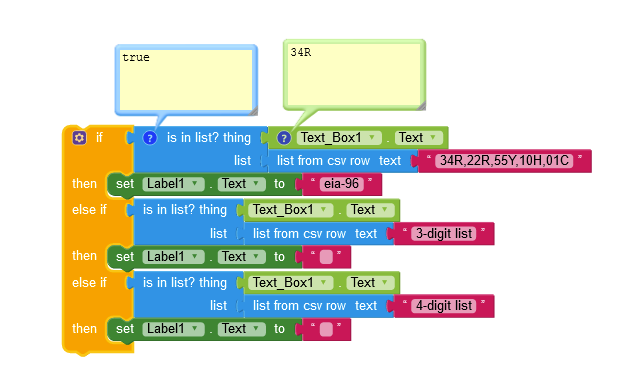# How to get if any number contains a variable from a list?

I want to draw a action if any number of code contains a variable from a list so, how can i do that.
example - 34R,22R,55Y,10H,01C
I have tried a code but that only excutes when there is only the alphebet, so help me with that.

sorry, I did not understand the example, you might want to elaborate…
Taifun

this are the codes of smd resistor eia-96 series, I have the code which is working for calculating the resistance but the thing is that smd also have codes that contains only numbers ex-102,220,1002,etc.so , I just need to identify if the code is of eia-96 series of different , so I need the way I can identify if the input by the user also contains number with some alphabet like R,C,H,Y etc.